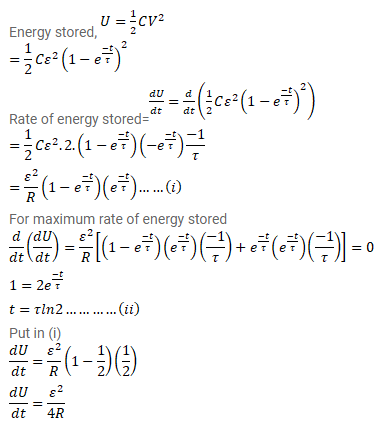# A capacitor of capacitance C is connected to a battery of

Question:

A capacitor of capacitance $C$ is connected to a battery of emf $\varepsilon$ at $t=0$ through a resistance $R$. Find the maximum rate at which energy is stored in the capacitor. When the rate doeshave this maximum value?

Solution: